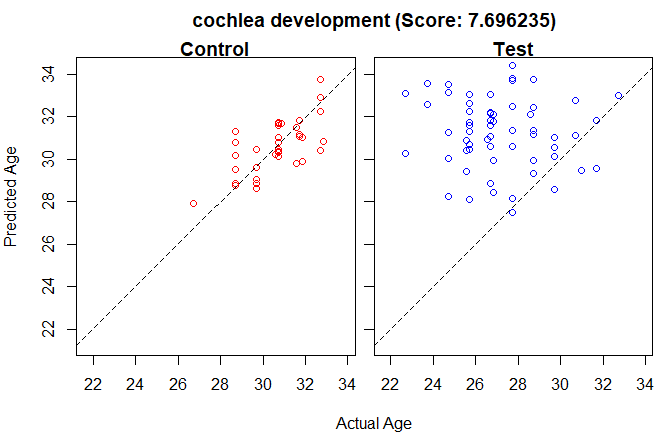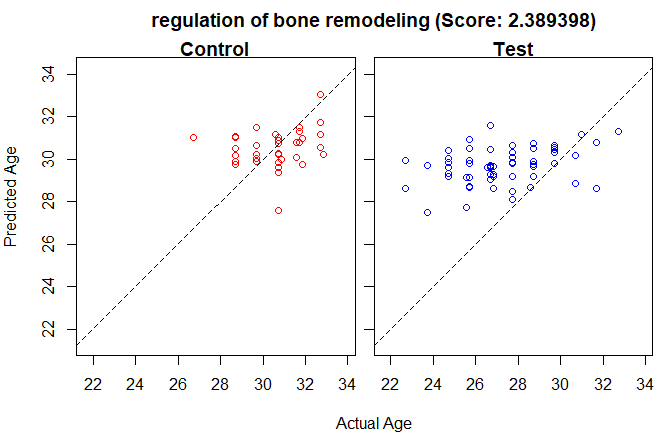# Getting Started with TEMPO

## Introduction

TEMPO is a pathway-based outlier detection approach for finding pathways showing significant temporal changes in expression patterns (or other real-valued genomic data), where each sample has meta-data corresponding to a temporal variable such as age or time. TEMPO was initially designed to identify functional developmental expression patterns that change in disease states (Pietras, et al., ACM-BCB, 2018).

TEMPO requires, as input, both a gene expression or other genomic data set with temporal meta-data, and a collection of gene sets, where gene names correspond to the gene names in the expression data set. There should also be phenotypic information about the samples, with some samples designated as controls and others as cases (sometimes called “test” samples below).

## Example Data

Example data is included with the package.

To get started, type the following commands:

library("tempoR")
data("dflatExample")
data("gse32472Example")

The first line loads the package. The second and third create example data objects. The variable “dflatExample” contains a small gene set collection: specifically, a subset of the Gene Ontology biological process gene sets, augmented with additional annotation around developmental processes for the DFLAT project (Wick, et al., BMC Bioinformatics, Feb 7;15:45, 2014).

The variable gse32472Example is a TEMPO data object containing a small fraction of published gene expression data from blood of infants born preterm (Pietrzyk, et al., PLoS One, Oct 23;8(10):e78585, 2013). One of the phenotypes of interest is whether the subjects developed bronchopulmonary dysplasia (BPD), a pulmonary complication sometimes resulting from very preterm birth. The full expression data set is available in GEO (https://www.ncbi.nlm.nih.gov/gds) under accession number GSE32472.

Each of the example data sets contain data as it would be read in from .gct, .cls, and .gmt files using the “loadCLS”, “loadGMT”, and “loadGCT” functions. The corresponding text files are also distributed with the package, and the example datasets could be created with the following set of commands:

dflatExample = loadGMT(paste0(path.package("tempoR"),"/dflatExample.gmt"))
gse32472Example = list()
gse32472Example$data = loadGCT(paste0(path.package("tempoR"),"/gse32472Example.gct")) gse32472Example$age = loadCLS(paste0(path.package("tempoR"),"/gse32472Example.age.cls"),
sampleNames=rownames(gse32472Example$data)) gse32472Example$bpd = loadCLS(paste0(path.package("tempoR"),"/gse32472Example.phen.cls"),
sampleNames=rownames(gse32472Example$data)) gse32472Example$ctrl = names(which(gse32472Example$bpd=="no")) gse32472Example$test = names(which(gse32472Example$bpd!="no")) ## Running TEMPO The core function for running TEMPO is called “tempo.run”. Results are reported for all gene sets meeting the desired significance and TEMPO score cutoffs. If the optional “output” argument is included (in the example below it is set to a temporary file, which should be replaced as appropriate), TEMPO produces output files “X.table” and “X.pdf,” where X is the string returned by the tempfile() call. The former is a table (tab-delimited text) of the reported gene sets and characteristics, and the latter contains scatterplots for all reported gene sets. A gene set is reported only if it has a raw p-value no larger than pCutoff, FDR q-value no larger than fdrCutoff, and a p-value associated with the control mean squared error no greater than pMseCutoff. For this example, we run only 4 permutations instead of the default 500, and use only 2 CPU cores instead of the default 24. Since the default reporting cutoffs are not meaningful with only 4 permutations, in this example, we report all results by setting all of the p-value, FDR, and MSE p-value cutoffs to 1, 2, and 1 respectively. results = tempo.run(phen=gse32472Example$bpd,
genesets=dflatExample,
X=gse32472Example$data, Y=gse32472Example$age,
numPerms=4,
nCores=2,
output=tempfile(tmpdir = tempdir()),
pCutoff=1,
fdrCutoff=2,
pMseCutoff = 1)

Above, TEMPO assumes the first entry in the list passed the “phen” is a control sample and all other types are test samples. The control and text samples can instead by specified exactly - in this case, the below produces the exact same results as the above:

results = tempo.run(ctrl=gse32472Example$ctrl, test=gse32472Example$test,
genesets=dflatExample,
X=gse32472Example$data, Y=gse32472Example$age,
numPerms=4,
nCores=42
output=tempfile(tmpdir = tempdir()),
pCutoff=1,
fdrCutoff=2,
pMseCutoff = 1)

## Viewing Output

Either of the previous commands will generate at .table and .pdf output file, which will look similar to the ones presented here.

The .table file is a tab delimited text file containing full information for all reported gene sets - which, for the purposes of this example, is all gene sets - ordered by decreasing TEMPO score. ctrlMSE is the mean squared error on the control samples; score refers to the TEMPO score; pMSE is the p-value associated with the control mean squared error; p is the raw p-value associated with the TEMPO score, and BH refers to the Benjamini-Hochberg adjusted false discovery rate associated with the TEMPO score.

ctrlMSE score pMSE p BH
positive regulation of immunoglobulin production 1.094505 7.746663 0.2 0.2 0.4000000
cochlea development 1.190724 7.696235 0.2 0.2 0.4000000
regulation of immunoglobulin production 1.339489 6.671197 0.2 0.2 0.4000000
response to cAMP 1.263533 6.238600 0.2 0.2 0.4000000
cerebral cortex radial glia guided migration 1.618526 6.218289 0.2 0.2 0.4000000
neural crest cell differentiation 1.747706 3.569756 0.4 0.2 0.4000000
positive regulation of execution phase of apoptosis 2.270780 3.446171 0.6 0.4 0.6666667
prostanoid biosynthetic process 1.420159 3.301954 0.2 0.2 0.4000000
regulation of endoplasmic reticulum unfolded protein response 2.091081 3.168399 0.4 0.2 0.4000000
cholesterol biosynthetic process 2.373395 3.071002 0.6 0.2 0.4000000
hormone metabolic process 1.748454 2.958436 0.2 0.2 0.4000000
negative regulation of intrinsic apoptotic signaling pathway in response to DNA damage 2.602118 2.886301 1.0 0.6 0.7500000
protein targeting to plasma membrane 2.470136 2.825820 0.6 0.6 0.7500000
detection of bacterium 1.980796 2.806186 0.6 0.6 0.7500000
neural precursor cell proliferation 1.929966 2.729198 0.2 0.6 0.7500000
positive regulation of branching involved in ureteric bud morphogenesis 2.226511 2.602062 0.4 0.8 0.8421053
pattern recognition receptor signaling pathway 2.482028 2.595295 0.8 0.4 0.6666667
negative regulation of multi-organism process 2.324547 2.593924 0.8 0.8 0.8421053
histone H3-K9 methylation 2.164172 2.562543 0.8 0.8 0.8421053
regulation of bone remodeling 2.315838 2.389398 0.4 1.0 1.0000000

The .pdf file contains plots for each reported gene set of the actual age for each sample vs. the age predicted by the PLS models inside TEMPO for that gene set. The command “tempo.mkplot” can be used to generate the plot for a specified gene set. “Cochlea development” is a gene set that is significant in the full anaylsis, while “regulation of bone remodeling” is not significant.

tempo.mkplot(results,"cochlea development")tempo.mkplot(results,"regulation of bone remodeling")## Additional TEMPO features

Instead of training and evaluating PLSR models on the control samples in leave-one-out cross-validation, models can instead be trained on a held-out set of training samples and evaluated scores on a separate set of control samples. The below example trains TEMPO models on the first 10 control samples, and calculates TEMPO scores using the second 10 control samples and all test samples:

results2 = tempo.run(train=gse32472Example$ctrl[1:10], ctrl=gse32472Example$ctrl[11:20],
test=gse32472Example$test, genesets=dflatExample, X=gse32472Example$data,
Y=gse32472Example\$age,
numPerms=4,
nCores=4)